Drop a Query

# Statistics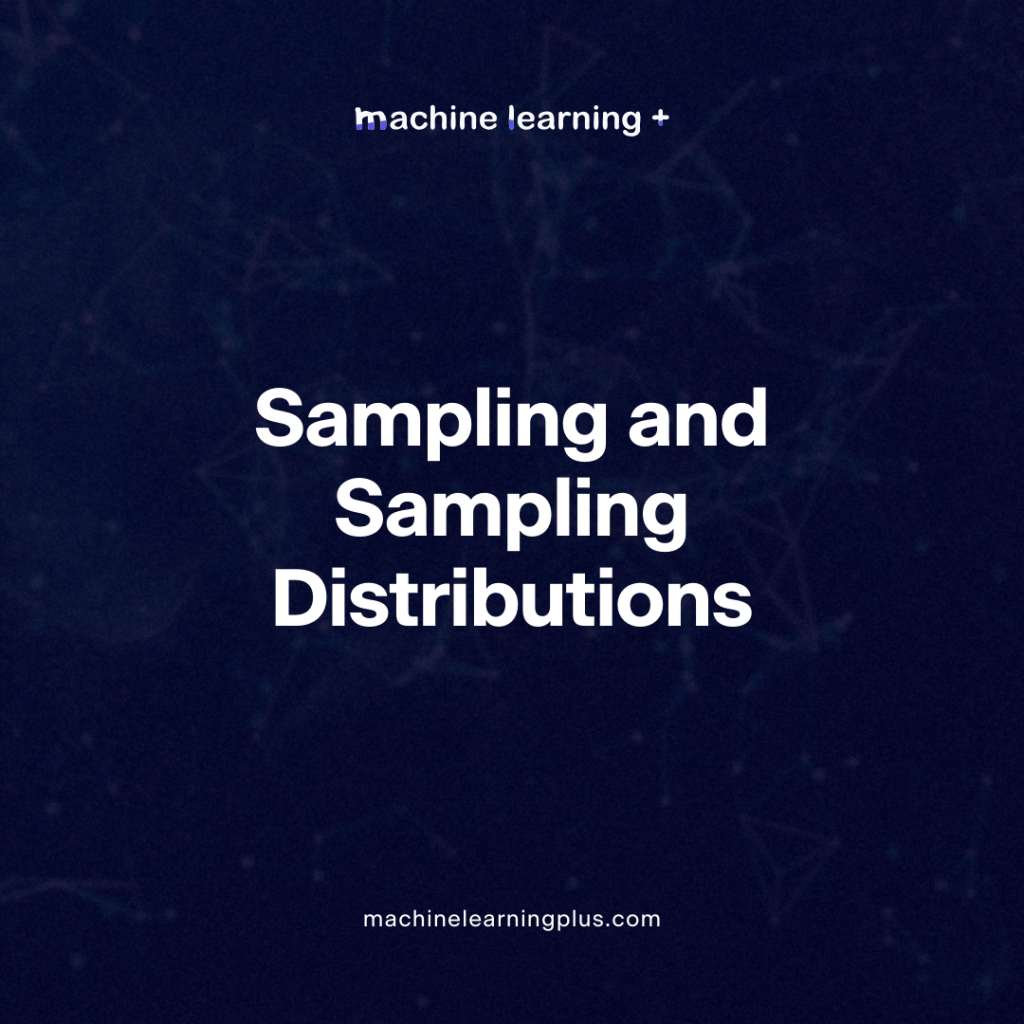## Sampling and Sampling Distributions – A Comprehensive Guide on Sampling and Sampling Distributions

Explore the fundamentals of sampling and sampling distributions in statistics. Dive deep into various sampling methods, from simple random to stratified, and uncover the significance of sampling distributions in detail. In this blog post we will learn What is Sampling? Why Sample? Types of Sampling Methods 3.1. Simple Random Sampling (SRS) 3.2. Stratified Sampling 3.3. …## Law of Large Numbers – A Deep Dive into the World of Statistics

The Law of Large Numbers (LLN) is a fundamental theorem in probability and statistics, serving as the basis for many concepts and practices in the field. If you’ve ever heard the saying “the more the better,” you can think of LLN as the mathematical rendition of this proverb. In this blog post, we’ll dive into …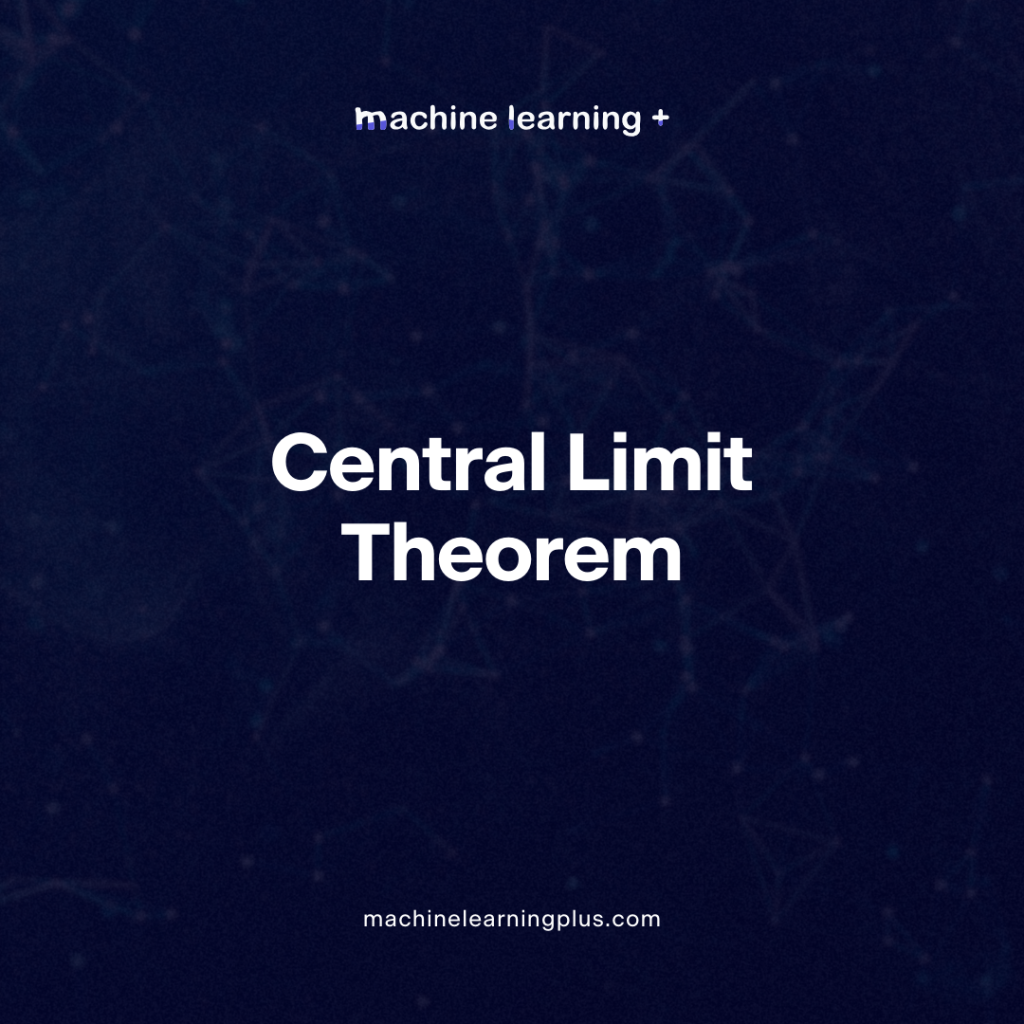## Central Limit Theorem – A Deep Dive into Central Limit Theorem and its Significance in Statistics

Statistics offers a vast array of principles and theorems that are foundational to how we understand data. Among them, the Central Limit Theorem (CLT) stands as one of the most important. Let’s dive deeper into the concept, ensuring that all points are covered and clarified. In this blog post we will learn: Simple Explanation of …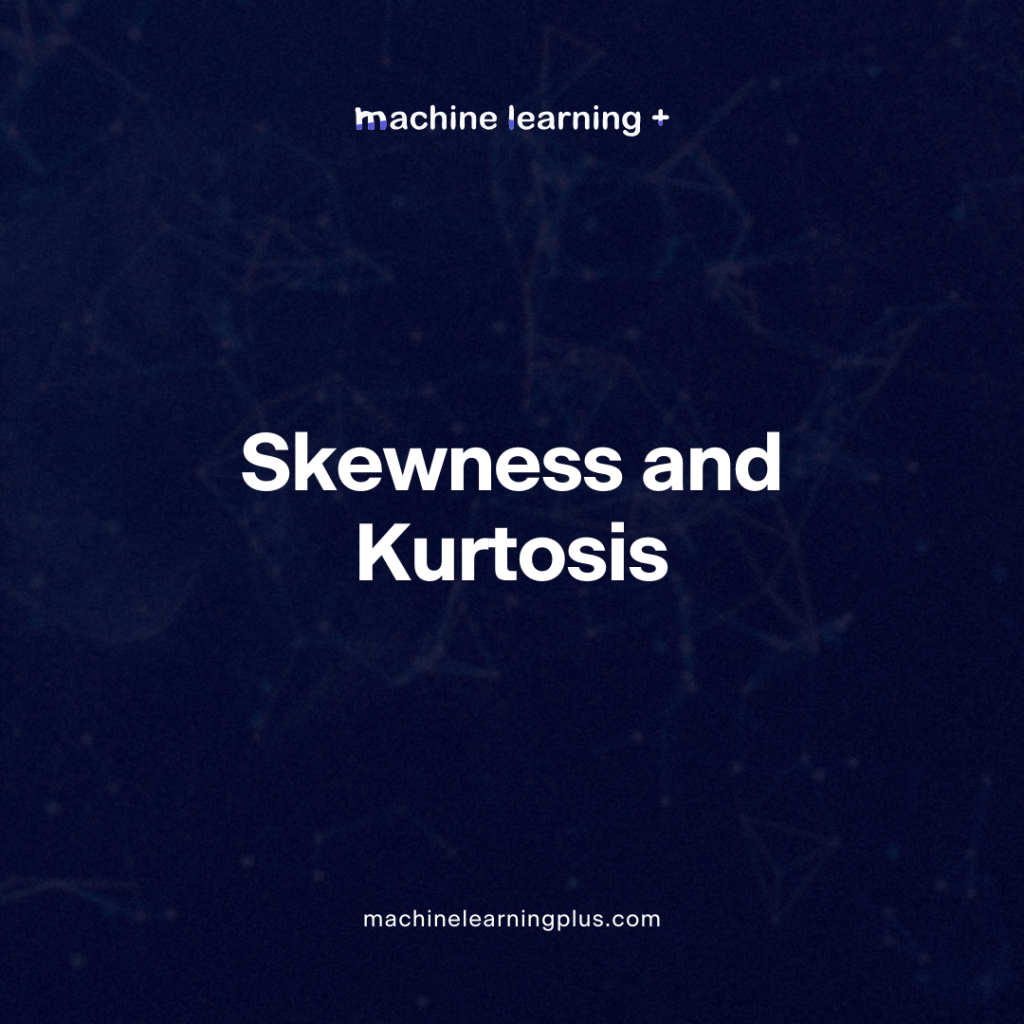## Skewness and Kurtosis – Peaks and Tails, Understanding Data Through Skewness and Kurtosis”

Statistics has a variety of tools to help us understand and interpret data. Two such tools are skewness and kurtosis, which give us insights into the shape of a data distribution. Let’s dive deeper into these concepts and understand their significance. In this blog post we will learn Skewness 1.1. Types of Skewness: 1.2. Rules …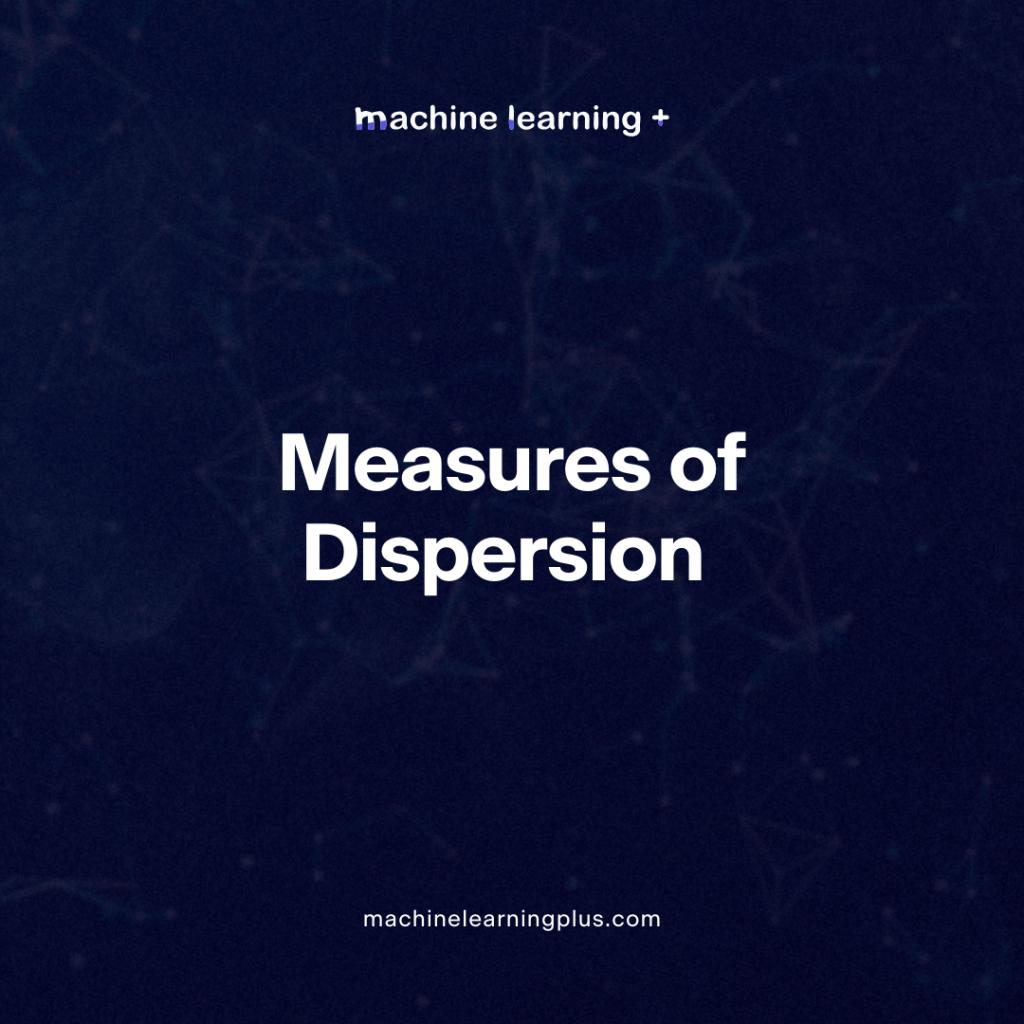## Measures of Dispersion – Unlocking the Variability Diving Deep into Measures of Dispersion

Dive deep into the world of statistics and measures of dispersion, from understanding its essence to its practical application using Python. In this Blog post we will learn: What is Dispersion in Statistics? Advantages and Applications of Measures of Dispersion: Types of Measures of Dispersion 3.1. Absolute Measure of Dispersion 3.2. Relative Measure of Dispersion …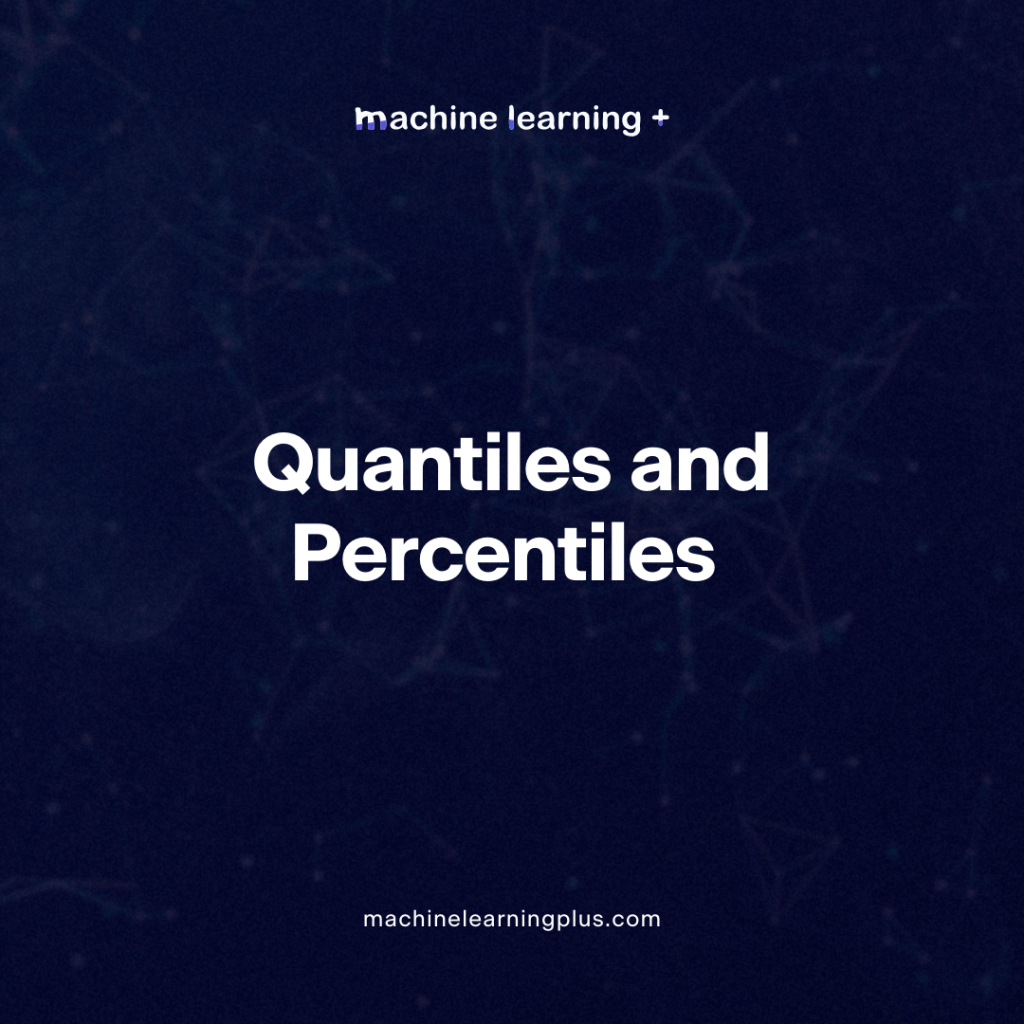## Quantiles and Percentiles – Understanding Quantiles and Percentiles, A Deep Dive with Python Examples

Quantiles and percentiles are crucial statistical concepts that assist in understanding and interpreting data. They are essentially tools to help divide datasets into smaller parts or intervals based on the data’s distribution. Let’s delve deep into these concepts and see them in action with Python. In this blog post we will learn Quantiles Percentiles Why …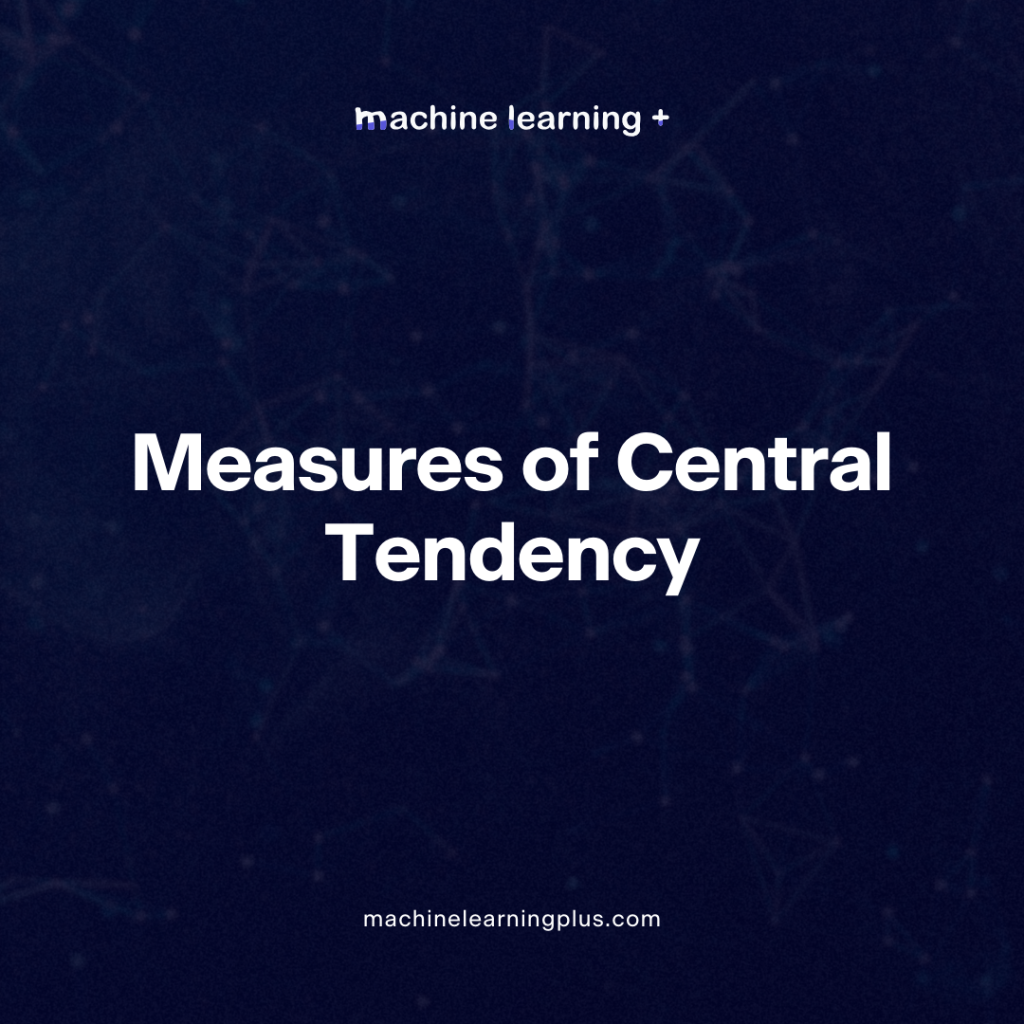## Measures of Central Tendency – A Clear Guide with Examples on Measures of Central Tendency

When diving into the world of statistics, you’ll frequently come across the term “measures of central tendency”. But what exactly does it mean, and why is it so important? Let’s break it down, step by step, with practical examples to drive the point home. In this blog post we will learn: What Are Measures of …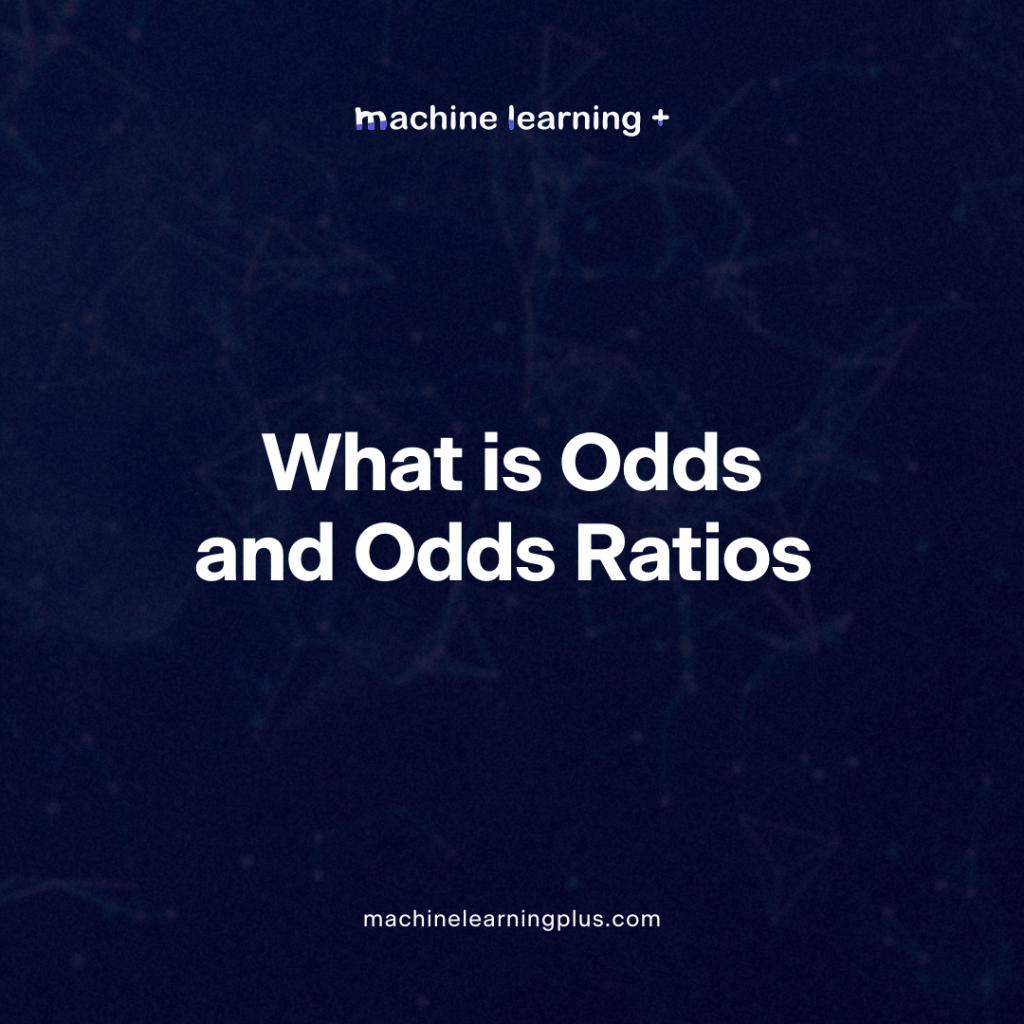## Odds and Odds Ratios – Understanding Odds and Odds Ratios in the World of Data Science

Probability, as a concept, plays an instrumental role in the world of data science. When we talk about probability, we’re essentially talking about quantifying the uncertainty or the chance of an event occurring. One term that often finds its way in probability is ‘odds’. Odds can be somewhat counterintuitive, especially for those who are familiar …## PySpark Outlier Detection and Treatment – A Comprehensive Guide How to handle Outlier in PySpark

Let’s dive deep into how to identify and treat outliers in PySpark, a popular open-source, distributed computing system that provides a fast and general-purpose cluster-computing framework for big data processing. Outliers are unusual data points that do not follow the general trend of a dataset. They can heavily influence the results of data analysis, predictive …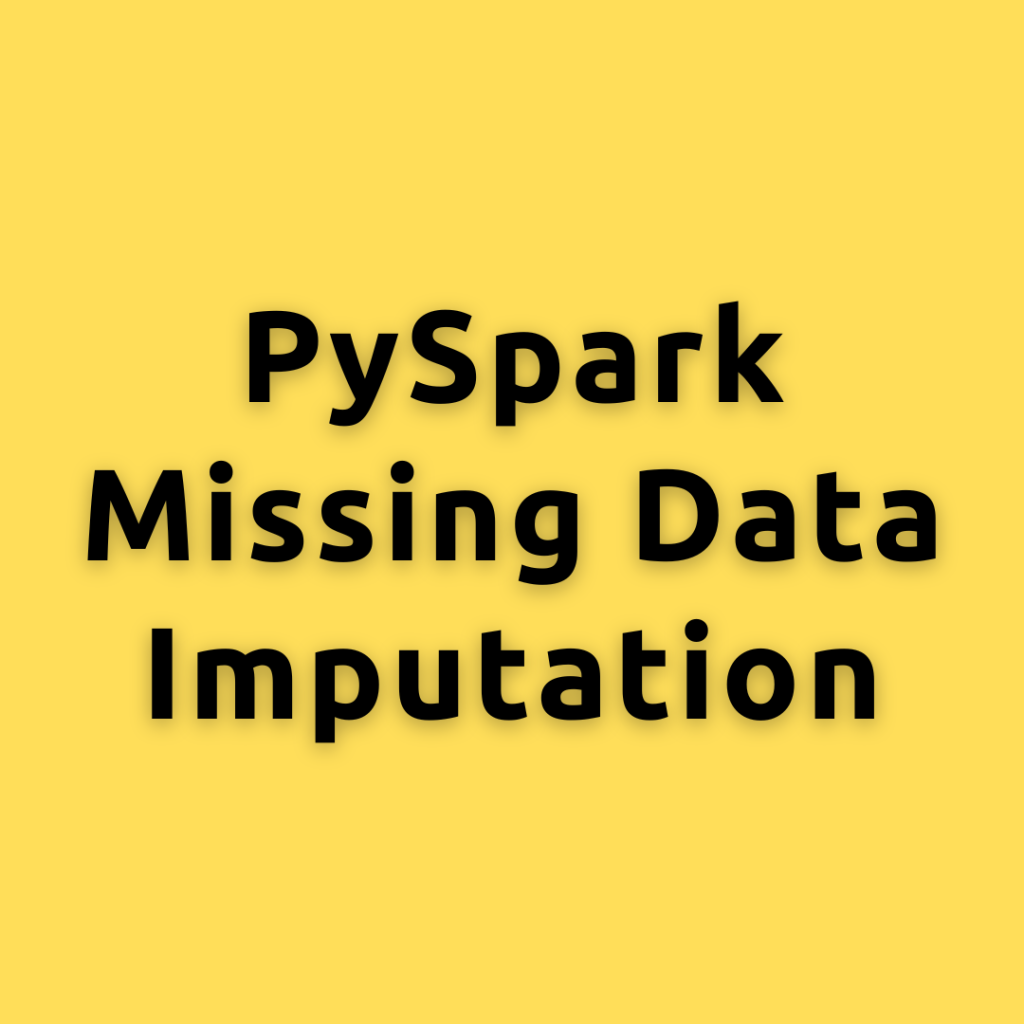## PySpark Missing Data Imputation – How to handle missing values in PySpark

Handling missing data is an essential step in the data preprocessing pipeline. let’s explore various methods to impute missing values in PySpark, a popular distributed data processing framework. We will discuss different techniques, such as mean, median, mode imputation, and using machine learning algorithms to fill in missing values. By the end of this post, …## PySpark Chi-Square Test – Understanding Chi-Square Test a Deep Dive with PySpark

Let’s explore the uses of Chi-Square in statistics and machine learning, and then demonstrate how to calculate the Chi-Square statistic in PySpark in different ways. Let’s dive into the world of statistics and machine learning, focusing on the Chi-Square Test. This statistical test is an essential tool for many data-driven applications and is widely used …## PySpark Statistics Variance – Understanding Variance a Deep Dive with PySpark

Let’s dive into the concept Variance, the formula to calculate Variance, and how to compute in PySpark, a powerful open-source data processing engine. When analyzing data, it’s essential to understand the underlying concepts of variability and dispersion. Two key measures for this are variance What is Variance? Variance is a measure of dispersion in a …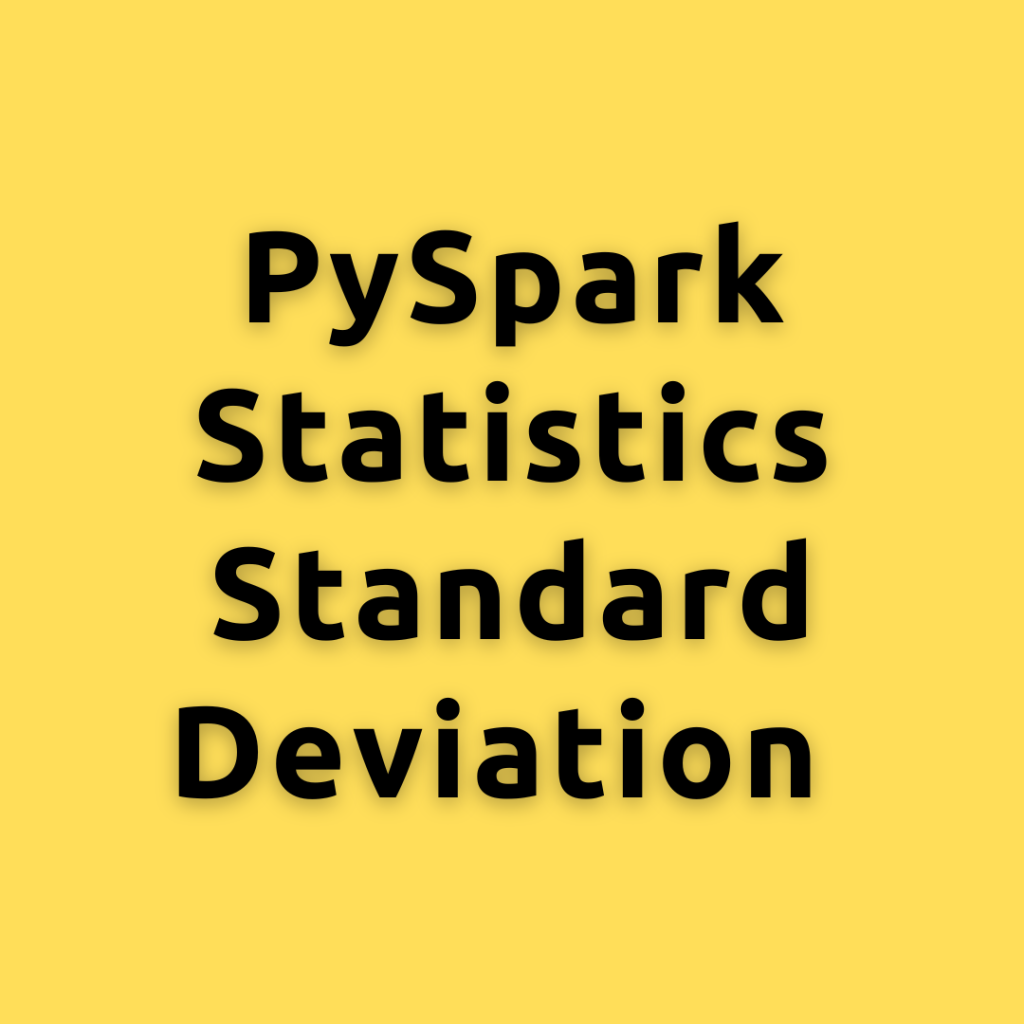## PySpark Statistics Standard Deviation – Calculating the Standard Deviation in PySpark a Comprehensive Guide for Everyone

Lets dive into the concept of Standard Deviation, its importance in statistics and machine learning, and explore different ways to calculate it using PySpark How to Calcualte Standard Deviation? Standard Deviation is a measure that quantifies the amount of variation or dispersion in a set of data values. It helps in understanding how far individual …

## Regression Coefficient Formula

Let’s understand the formula for the linear regression coefficients. That is the formula for both alpha and the beta. Now, if you have simple linear regression that does, you have just 1x variable in your data, you will be able to compute the values of alpha and beta using this formula. Let’s suppose you …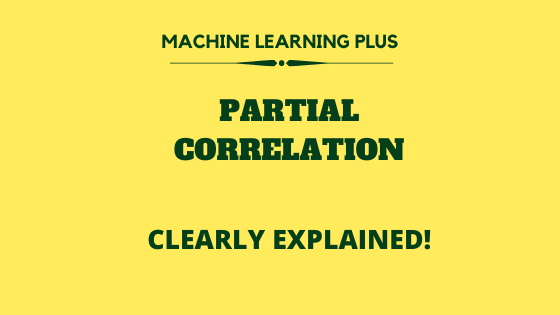## Partial Correlation

What is Partial Correlation and it’s purpose Partial correlation is used to find the correlation between two variables (typically a dependent and an independent variable) with the effect of other influencing variables being controlled. For example, if there are three variables ‘A’, ‘B’, ‘Z’, If you want to find the relationship between ‘A’ and ‘B’ …## Chi-Square test – How to test statistical significance for categorical data?

What is chi-square test and its purpose? Chi-square test was invented in the year ‘1900’ by the revered mathematician ‘Karl Pearson’. Chi-square test, also written as χ2 test is used to determine whether there is a statistically significant difference between the observed frequency and the expected frequency in one or more categories of the contingency …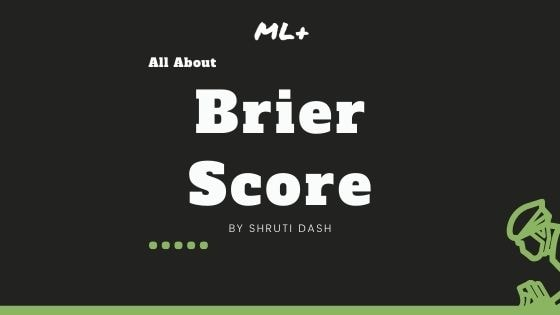## Brier Score – How to measure accuracy of probablistic predictions

Brier score is an evaluation metric that is used to check the goodness of a predicted probability score. This is very similar to the mean squared error, but only applied for prediction probability scores, whose values range between 0 and 1. Overview In this tutorial, you will understand: What is Brier score? How is Brier …

## One Sample T Test – Clearly Explained with Examples | ML+

One sample T-Test tests if the given sample of observations could have been generated from a population with a specified mean. If it is found from the test that the means are statistically different, we infer that the sample is unlikely to have come from the population. For example: If you want to test a …

## Standard Error in Statistics – Understanding the concept, formula and how to calculate

Standard error of the mean measures how spread out the means of the sample can be from the actual population mean. Standard error allows you to build a relationship between a sample statistic (computed from a smaller sample of the population) and the population’s actual parameter. Standard Error – A practical guide with examples. Photo …

## Confidence Interval in Statistics – Formula and Mathematical Calculation

Confidence interval is a measure to quantify the uncertainty in an estimated statistic (like the mean) when the true population parameter is unknown. Training Custom Text Classification Model in spaCy. Photo by Jessica Wong. You will know 1. What is Confidence Interval? 2. Two types of Confidence Intervals problems 3. Difference between Population parameter vs …Course Preview

## Machine Learning A-Z™: Hands-On Python & R In Data Science

### Free Sample Videos:#### Machine Learning A-Z™: Hands-On Python & R In Data Science#### Machine Learning A-Z™: Hands-On Python & R In Data Science#### Machine Learning A-Z™: Hands-On Python & R In Data Science#### Machine Learning A-Z™: Hands-On Python & R In Data Science